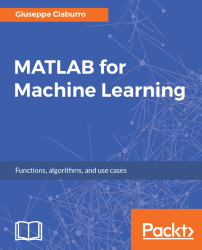•#### MATLAB for Machine Learning#### Overview of this book

MATLAB is the language of choice for many researchers and mathematics experts for machine learning. This book will help you build a foundation in machine learning using MATLAB for beginners. You’ll start by getting your system ready with t he MATLAB environment for machine learning and you’ll see how to easily interact with the Matlab workspace. We’ll then move on to data cleansing, mining and analyzing various data types in machine learning and you’ll see how to display data values on a plot. Next, you’ll get to know about the different types of regression techniques and how to apply them to your data using the MATLAB functions. You’ll understand the basic concepts of neural networks and perform data fitting, pattern recognition, and clustering analysis. Finally, you’ll explore feature selection and extraction techniques for dimensionality reduction for performance improvement. At the end of the book, you will learn to put it all together into real-world cases covering major machine learning algorithms and be comfortable in performing machine learning with MATLAB.
Title PageCreditsForewordwww.PacktPub.comCustomer FeedbackPrefaceFree Chapter
Getting Started with MATLAB Machine LearningImporting and Organizing Data in MATLABFrom Data to Knowledge DiscoveryFinding Relationships between Variables - Regression TechniquesPattern Recognition through Classification AlgorithmsIdentifying Groups of Data Using Clustering MethodsSimulation of Human Thinking - Artificial Neural NetworksImproving the Performance of the Machine Learning Model - Dimensionality ReductionMachine Learning in Practice## Chapter 5. Pattern Recognition through Classification Algorithms

Classification algorithms study how to automatically learn to make accurate predictions based on observations. Starting from a set of predefined class labels, the algorithm gives each piece of data input a class label in accordance with the training model. If there are just two distinction classes, we talk about binary classification; otherwise, we go for multi-class classification. In more detail, each category corresponds to a different label; the algorithm attaches a label to each instance, which simply indicates which class the data belongs to. A procedure that can perform this function is commonly called a classifier.

Classification has some analogy with regression, which we studied in Chapter 4, Finding Relationships between Variables - Regression Techniques. As well as regression, classification uses known labels of a training dataset to predict the response of the new test dataset. The main difference between regression...